Next: Hydrostatic Equilibrium of Atmosphere Up: Classical Thermodynamics Previous: Calculation of Specific Heats

Suppose that the temperature of an ideal gas is held constant by keeping the gas in thermal contact with a heat reservoir. If the gas is allowed to expand quasi-statically under these so-called isothermal conditions then the ideal gas equation of state tells us that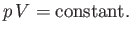(6.52)

This result is known as the isothermal gas law.

Suppose, now, that the gas is thermally isolated from its surroundings. If the gas is allowed to expand quasi-statically under these so-called adiabatic conditions then it does work on its environment, and, hence, its internal energy is reduced, and its temperature changes. Let us calculate the relationship between the pressure and volume of the gas during adiabatic expansion. According to the first law of thermodynamics,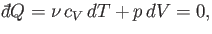(6.53)

in an adiabatic process (in which no heat is absorbed). [See Equation (6.35).] The ideal gas equation of state, (6.10), can be differentiated, yielding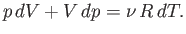(6.54)

The temperature increment,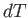, can be eliminated between the previous two expressions to give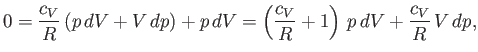(6.55)

which reduces to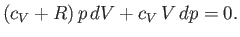(6.56)

Dividing through by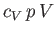yields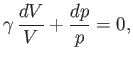(6.57)

where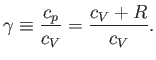(6.58)

It turns out that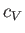is a slowly-varying function of temperature in most gases. Consequently, it is usually a good approximation to treat the ratio of specific heats,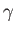, as a constant, at least over a limited temperature range. Ifis constant then we can integrate Equation (6.57) to give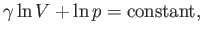(6.59)

or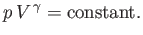(6.60)

This result is known as the adiabatic gas law. It is straightforward to obtain analogous relationships betweenand, and betweenand, during adiabatic expansion or contraction. In fact, because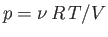, the previous formula also implies that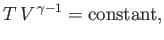(6.61)

and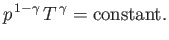(6.62)

Equations (6.60)-(6.62) are all completely equivalent.Next: Hydrostatic Equilibrium of Atmosphere Up: Classical Thermodynamics Previous: Calculation of Specific Heats
Richard Fitzpatrick 2016-01-25